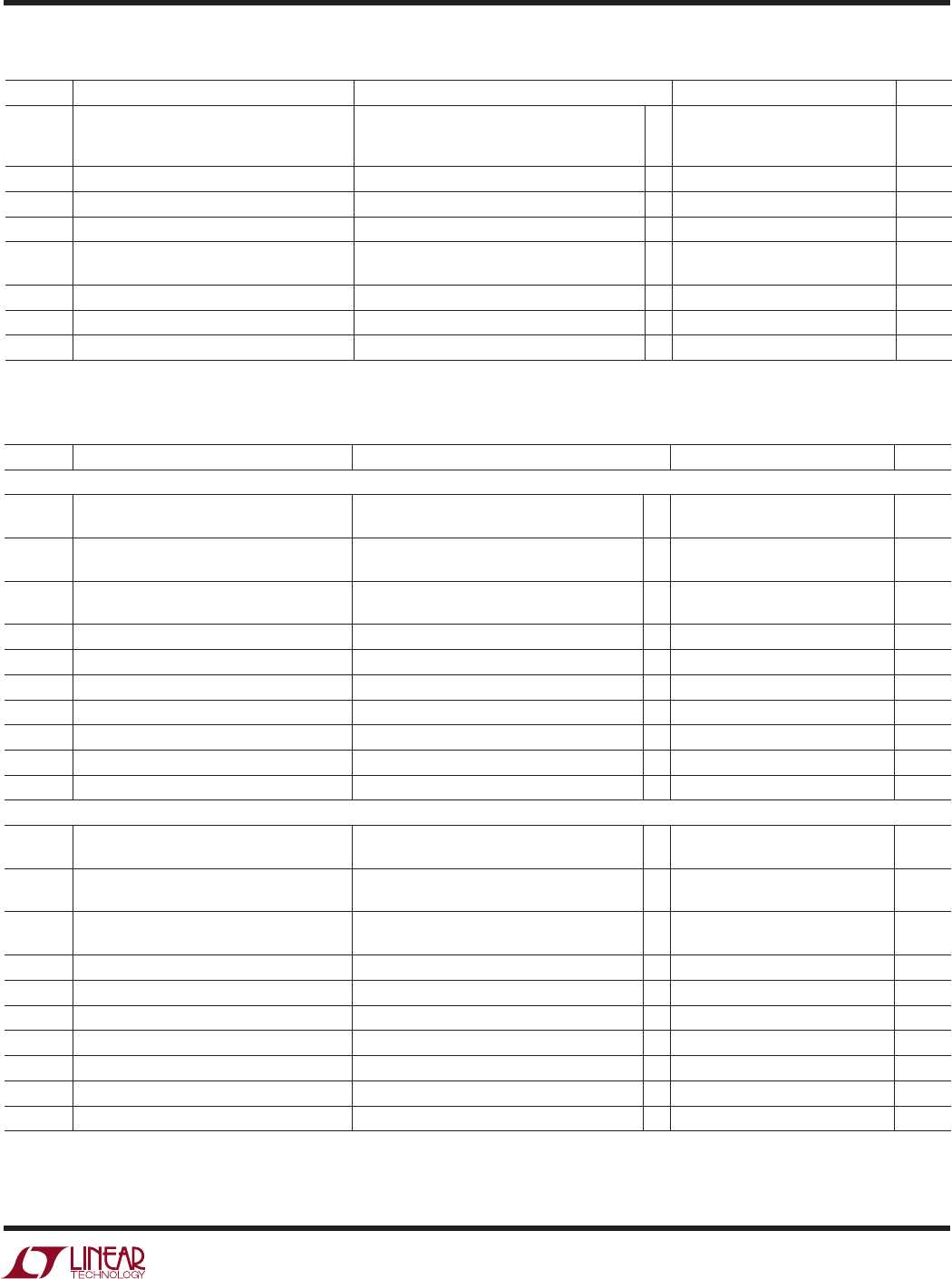# Datasheet3
LTC1688/LTC1689
16889fa
SYMBOL PARAMETER CONDITIONS MIN TYP MAX UNITS
∆V
OC
Change in Magnitude of Driver Common R = 25 or 50, Figure 1 0.1 V
Mode Output Voltage for Complementary
Output States
V
IH
Input High Voltage EN, ENB, EN12, EN34, DI 1.4 V
V
IL
Input Low Voltage EN, ENB, EN12, EN34, DI 0.5 V
I
IN1
Input Current EN, ENB, EN12, EN34, DI (Note 3) ±1 µA
I
OZ
Three-State (High Impedance) V
OUT
= –7V to 10V (Note 3) ±1 ±200 µA
Output Current
I
DD
Supply Current of Entire Device No Load, Digital Input Pins = 0V or V
DD
5mA
I
OSD1
Driver Short-Circuit Current, V
OUT
= HIGH V
OUT
= –7V to 8V (Note 3) ±20 mA
I
OSD2
Driver Short-Circuit Current, V
OUT
= LOW V
OUT
= –7V to 8V (Note 3) ±20 mA
Note 1: Absolute Maximum Ratings are those values beyond which the life
of a device may be impaired.
Note 2: All currents into the device pins are positive; all currents out of the
device pins are negative.
Note 3: Guaranteed by design or correlation, but not tested.
SWITCHING CHARACTERISTICS
U
SYMBOL PARAMETER CONDITIONS MIN TYP MAX UNITS
V
DD
= 5V, T
A
= 25
°
C, Unless Otherwise Noted (Note 2)
t
PLH
, t
PHL
Driver Input-to-Output Propagation Delay R
DIFF
= 50, C
L1
= C
L2
= 25pF, 4812 ns
Figures 2, 4
t
SKEW
Driver Output-to-Output Skew R
DIFF
= 50, C
L1
= C
L2
= 25pF, 500 ps
Figures 2, 4
t
r
, t
f
Driver Rise/Fall Time R
DIFF
= 50, C
L1
= C
L2
= 25pF, 2 ns
Figures 2, 4
t
ZH
Driver Enable to Output High C
L
= 25pF, S2 Closed, Figures 3, 5 10 35 ns
t
ZL
Driver Enable to Output Low C
L
= 25pF, S1 Closed, Figures 3, 5 10 35 ns
t
LZ
Driver Disable from Low C
L
= 15pF, S1 Closed, Figures 3, 5 25 65 ns
t
HZ
Driver Disable from High C
L
= 15pF, S2 Closed, Figures 3, 5 25 65 ns
C
L(MAX)
Maximum Output Capacitive Load (Note 3) 200 pF
Maximum Data Rate (Note 3) 100 Mbps
Maximum Driver Input Rise/Fall Time (Note 3) 500 ns
V
DD
= 3V, T
A
= 25
°
C, Unless Otherwise Noted (Note 2)
t
PLH
, t
PHL
Driver Input-to-Output Propagation Delay R
DIFF
= 50, C
L1
= C
L2
= 25pF, 11 ns
Figures 2, 4
t
SKEW
Driver Output-to-Output Skew R
DIFF
= 50, C
L1
= C
L2
= 25pF, 1 ns
Figures 2, 4
t
r
, t
f
Driver Rise/Fall Time R
DIFF
= 50, C
L1
= C
L2
= 25pF, 4 ns
Figures 2, 4
t
ZH
Driver Enable to Output High C
L
= 25pF, S2 Closed, Figures 3, 5 25 ns
t
ZL
Driver Enable to Output Low C
L
= 25pF, S1 Closed, Figures 3, 5 25 ns
t
LZ
Driver Disable from Low C
L
= 15pF, S1 Closed, Figures 3, 5 50 ns
t
HZ
Driver Disable from High C
L
= 15pF, S2 Closed, Figures 3, 5 50 ns
C
L(MAX)
Maximum Output Capacitive Load (Note 3) 200 pF
Maximum Data Rate 50 Mbps
Maximum Driver Input Rise/Fall Time (Note 3) 500 ns
DC ELECTRICAL CHARACTERISTICS
The denotes the specifications which apply over the full operating
temperature range, otherwise specifications are at T
A
= 25°C.
The denotes the specifications which apply over the full operating
temperature range, otherwise specifications are at T
A
= 25°C.